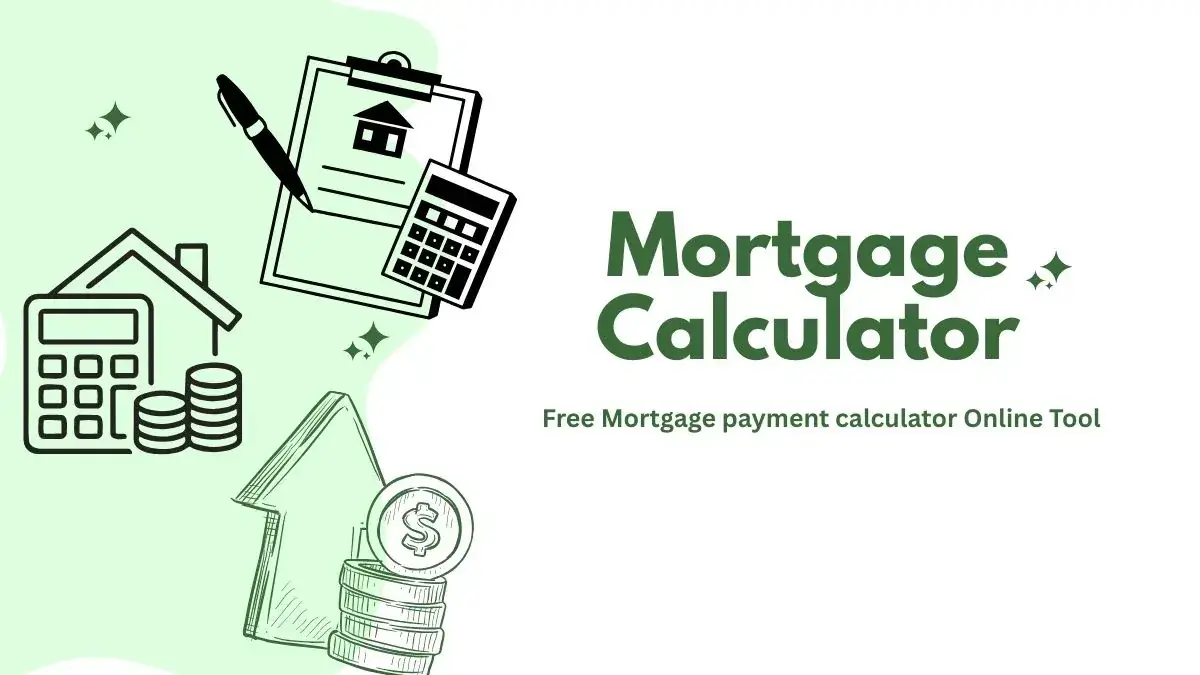# Mortgage Calculate.

Free mortgage calculator to find monthly payment, total home ownership cost, and amortization schedule with options for taxes, PMI, HOA, and early payoff.

Loan Amount
Percent %
years

Monthly EMI:

Principal amount:

Total interest:

Total amount:## What is Mortgage Loan?

If you take a loan on any property that you already have, then it is called Mortgage Loan. Mortgage loan is also known as Loan Against Property (LAP). This means that today we have a property. We mortgage it to the bank and we take the money, then it is called mortgage loan.

## Why mortgage loan is taken?

Mortgage loan is taken because the property we have, we take the money by mortgaging it to the bank.We can use it in any way like starting a new business, moving the business forward, setting up a factory, buying a masonry, you have to buy a plot. Whatever money you take from the mortgage loan, you can do anything with that money.

## How deos a Mortgage Loan calculator work?

A loan calculator work on the following formula.

M = [P x R x (1+R) ^N] / [(1+R) ^N-1]

In the above formula

 M Mortgage amount . P Principal amount. R Rate of interest. N Loan tenure.

For example, If a x person avails a loan of \$1000,000 at an annual interest rate of 6% for a tenure of 96 months (8 years), then his EMI will be calculated as under.

If rate of interest is 6% p.a. then r = 6/12/100 = 0.005.

EMI= 1000,000 * 0.005 * (1 + 0.005)120 / ((1 + 0.005)120 - 1) = 13,141.

The total amount payable will be \$13,141 * 96 = \$1,261,577. Principal loan amount is \$1000,000 and the Interest amount will be \$261,577.### Mortgage Loan Calculator Features.

Some of the features of the Mortgage calculator tool are as follows:

• In this, you can select different types of currency with the help of select box. Sorry if you don't have the currency of your country, if you want to add, then contact.
• For ease, you can easily increase or decrease the value with the help of slider on Principal Amount, Rate of interest, Loan tenure.
• In Return, we have told you with the help of Google chart, with the help of which you can easily know your return and your total interest.
• ###### FAQs
The Six Main Types of Mortgages?
1. Conventional Mortgages.
2. Conforming Mortgage Loans.
3. Nonconforming Mortgage Loans.
4. Government-Insured Federal Housing Administration (FHA) Loans.
5. Government-Insured Veterans Affairs (VA) Loans.
6. Government-Insured U.S. Department of Agriculture (USDA) Loans.
This information is taken from Investopedia.
How do I calculate my mortgage?
You can calculate mortgage with the help of this formula.
M = [P x R x (1+R) ^N] / [(1+R) ^N-1]
in which
M = Mortgage. P = Principal amount. R = Rate of interest. N = Loan tenure.